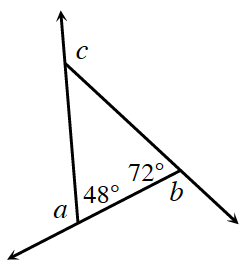### Home > INT2 > Chapter 8 > Lesson 8.1.1 > Problem8-14

8-14.

In the diagram at right, what are the measures of the exterior angles of the triangle, which are labeled $a, b,$ and $c$? What is the sum of the exterior angle measures?$m∠a + 48° = 180°$

Use similar methods to calculate $m∠b$ and $m∠c$.

$360°$